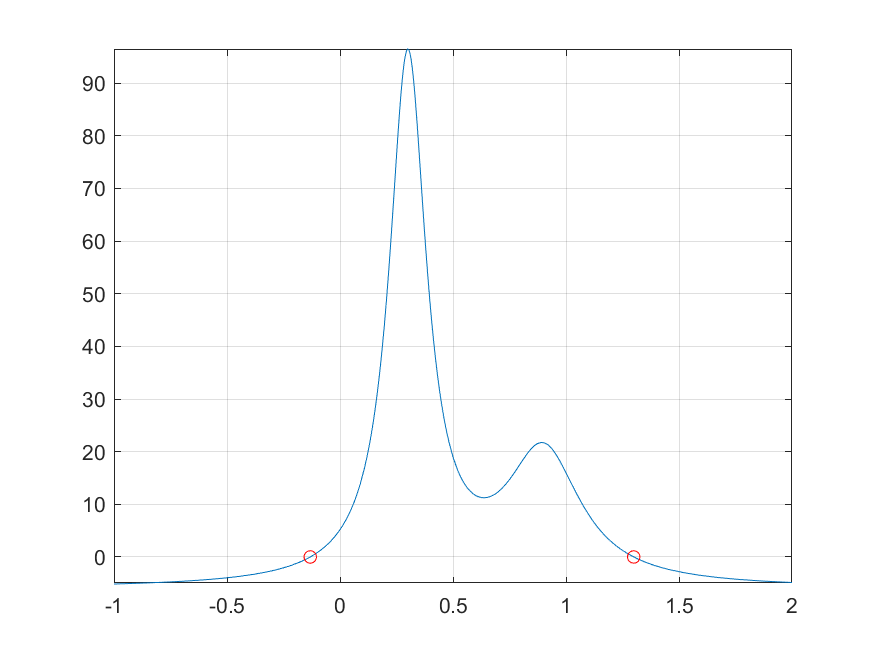## 8-3 嚙踝蕭嚙踝蕭嚙踝蕭嚙踝蕭嚙踝蕭嚙踝蕭嚙踝蕭嚙?

fzero 指令可用於單變數函數的求根，其使用語法如下：

x = fzero(fun, x0)

• 若 x0 為一個起始點，則 fzero 會自動找出附近包含零點（即根，或函數變號點）的區間，並逐步縮小此區間以找出零點。若 fzero 無法找出此區間，則傳回 NaN。
• 若已知使函數值不同號的兩點，則我們可以由 x0 直接指定尋根的區間，以使 fzero 更快速地找到位於此區間內的根。

Example 1: 08-一般數學函數的處理與分析/fzero01.mx = fzero(@humps, 1.5); % 求靠近 1.5 附近的根 y = humps(x); % 帶入求值 fprintf('humps(%f) = %f\n', x , y);humps(1.299550) = 0.000000

Example 2: 08-一般數學函數的處理與分析/fzero02.mx = fzero(@humps, [-1, 1]); % 求落於區間 [-1, 1] 的根 y = humps(x); % 帶入求值 fprintf('humps(%f) = %f\n', x , y);humps(-0.131618) = 0.000000

Example 3: 08-一般數學函數的處理與分析/fzero03.mfplot(@humps, [-1, 2]); grid on z1 = fzero(@humps, 1.5); z2 = fzero(@humps, [-1, 1]); line(z1, humps(z1), 'marker', 'o', 'color', 'r'); % 畫出第一個零點的位置 line(z2, humps(z2), 'marker', 'o', 'color', 'r'); % 畫出第二個零點的位置Example 4: 08-一般數學函數的處理與分析/fzero04.mopt = optimset('disp', 'iter'); % 顯示每個 iteration 的結果 a = fzero(@humps, [-1, 1], opt) Func-count x f(x) Procedure 2 -1 -5.13779 initial 3 -0.513876 -4.02235 interpolation 4 -0.513876 -4.02235 bisection 5 -0.473635 -3.83767 interpolation 6 -0.115287 0.414441 bisection 7 -0.115287 0.414441 interpolation 8 -0.132562 -0.0226907 interpolation 9 -0.131666 -0.0011492 interpolation 10 -0.131618 1.88371e-07 interpolation 11 -0.131618 -2.7935e-11 interpolation 12 -0.131618 8.88178e-16 interpolation 13 -0.131618 8.88178e-16 interpolation Zero found in the interval [-1, 1] a = -0.1316

Hint
• optimset 通常用於設定最佳化的選項，將在下節中詳細介紹。

MATLAB程式設計：進階篇